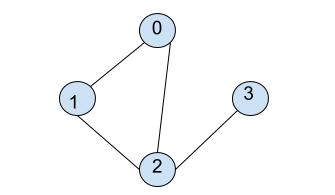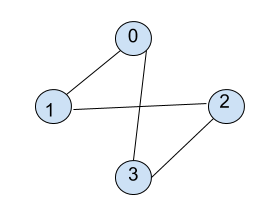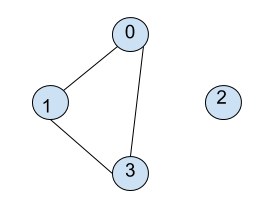6
Avg. time to solve
10 min
Success Rate
90%

Problem Statement

Note:

1. Here, you need to consider that you need to print the BFS path starting from vertex 0 only.
2. V is the number of vertices present in graph G, and all vertices are numbered from 0 to V-1.
3. E is the number of edges present in graph G.
4. Graph input is provided as the number of vertices and a list of edges.
5. Handle for Disconnected Graphs as well.

For Example: Consider graph:Here, starting from 0, it is connected to 1 and 2 so, BFS traversal from here will be [0, 1, 2 ]. Now, 3 is also connected to 2. So, BFS traversal becomes [0, 1, 2, 3].

Note:

For each node, the correct order of printing the connected nodes will be sorted order, i.e., if {3,6,9,4} are connected to 1, then the correct order of their printing is {1,3,4,6,9}.

Input Format :

The first line of input contains two integers that denote the value of V and E.
Each of the following E lines contains space-separated two integers that denote an edge between vertex A and B.

Output Format :

For each test case, print the BFS Traversal, as described in the task.
Output for each test case will be printed in a separate line.

Note:

You do not need to print anything; it has already been taken care of. Just implement the given function.

Constraints :

0 <= V <= 10^4
0 <= E <= (V * (V - 1)) / 2
0 <= A <= V - 1
0 <= B <= V - 1

Where 'V' is the number of vertices, 'E' is the number of edges, 'A' and 'B' are the vertex numbers.
Time Limit: 1 second
4 4
0 1
0 3
1 2
2 3
0 1 3 2
Explanation for sample input 1:Starting from 0, it is connected to 1 and 3, which will be printed. Then comes 2, which was connected to 1.
4 3
0 1
0 3
1 3
0 1 3 2
Explanation for sample input 2:Starting from 0, it is connected to 1 and 3, which will be printed. The remaining node is 2, which will be printed at the end.Console